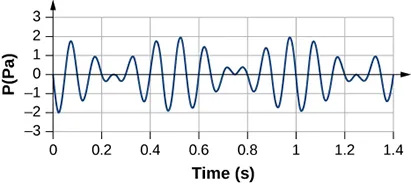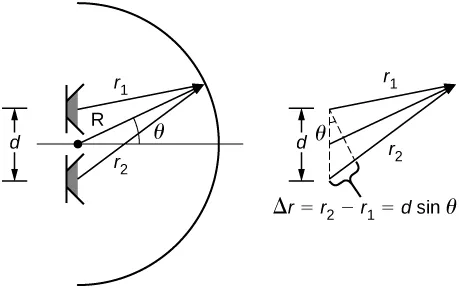University Physics Volume 1

# Challenge Problems

University Physics Volume 1Challenge Problems

### Challenge Problems

139.

Two sound speakers are separated by a distance d, each sounding a frequency f. An observer stands at one speaker and walks in a straight line a distance x, perpendicular to the the two speakers, until he comes to the first maximum intensity of sound. The speed of sound is v. How far is he from the speaker?

140.

Consider the beats shown below. This is a graph of the gauge pressure versus time for the position $x=0.00m.x=0.00m.$ The wave moves with a speed of $v=343.00m/s.v=343.00m/s.$ (a) How many beats are there per second? (b) How many times does the wave oscillate per second? (c) Write a wave function for the gauge pressure as a function of time.141.

Two speakers producing the same frequency of sound are a distance of d apart. Consider an arc along a circle of radius R, centered at the midpoint of the speakers, as shown below. (a) At what angles will there be maxima? (b) At what angle will there be minima?142.

A string has a length of 1.5 m, a linear mass density $μ=0.008kg/m,μ=0.008kg/m,$, and a tension of 120 N. If the air temperature is $T=22°C,T=22°C,$ what should the length of a pipe open at both ends for it to have the same frequency for the $n=3n=3$ mode?

143.

A string $(μ=0.006kgm,L=1.50m)(μ=0.006kgm,L=1.50m)$ is fixed at both ends and is under a tension of 155 N. It oscillates in the $n=10n=10$ mode and produces sound. A tuning fork is ringing nearby, producing a beat frequency of 23.76 Hz. (a) What is the frequency of the sound from the string? (b) What is the frequency of the tuning fork if the tuning fork frequency is lower? (c) What should be the tension of the string for the beat frequency to be zero?

144.

A string has a linear mass density $μμ$, a length L, and a tension of $FTFT$, and oscillates in a mode n at a frequency f. Find the ratio of $ΔffΔff$ for a small change in tension.

145.

A string has a linear mass density $μ=0.007kg/m,μ=0.007kg/m,$ a length $L=0.70m,L=0.70m,$ a tension of $FT=110N,FT=110N,$ and oscillates in a mode $n=3n=3$. (a) What is the frequency of the oscillations? (b) Use the result in the preceding problem to find the change in the frequency when the tension is increased by $1.00%1.00%$.

146.

A speaker powered by a signal generator is used to study resonance in a tube. The signal generator can be adjusted from a frequency of 1000 Hz to 1800 Hz. First, a 0.75-m-long tube, open at both ends, is studied. The temperature in the room is $TF=85.00°F.TF=85.00°F.$ (a) Which normal modes of the pipe can be studied? What are the frequencies and wavelengths? Next a cap is place on one end of the 0.75-meter-long pipe. (b) Which normal modes of the pipe can be studied? What are the frequencies and wavelengths?

147.

A string on the violin has a length of 23.00 cm and a mass of 0.900 grams. The tension in the string 850.00 N. The temperature in the room is $TC=24.00°C.TC=24.00°C.$ The string is plucked and oscillates in the $n=9n=9$ mode. (a) What is the speed of the wave on the string? (b) What is the wavelength of the sounding wave produced? (c) What is the frequency of the oscillating string? (d) What is the frequency of the sound produced? (e) What is the wavelength of the sound produced?

Order a print copy

As an Amazon Associate we earn from qualifying purchases.